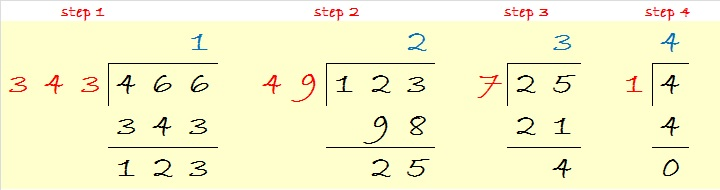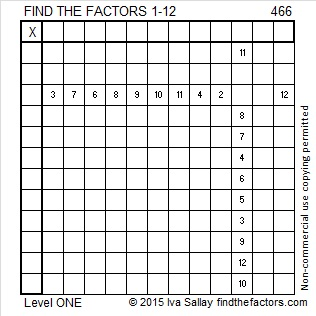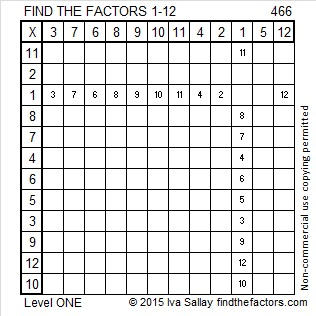# 466 and Level 1

466 is an interesting number whose factors are listed below today’s puzzle.

Thanks to OEIS.org, I know that 466 is 1234 in base 7. There are two different ways to change 466 from base 10 to base 7.

The first way gives the answer at the top of all the division problems and has you working from left to right. The division problems may be more difficult because division by 7 cubed and 7 squared are required, but the concept of what is happening is fairly easy to understand.For the second way, each of the division problems is quite easy to do. However working from right to left and finding the answer at the bottom of all the problems may be confusing to some people.Changing a number from base ten to base seven can be a bit of a challenge. However, today’s Find the Factors puzzle is as easy as they get. Every person who has learned how to multiply can find the factors for this puzzle and then make the puzzle work like a multiplication table:Print the puzzles or type the solution on this excel file:  12 Factors 2015-04-20

—————————————————————————————————

Here is the factoring information for 466:

• 466 is a composite number.
• Prime factorization: 466 = 2 x 233
• The exponents in the prime factorization are 1 and 1. Adding one to each and multiplying we get (1 + 1)(1 + 1) = 2 x 2 = 4. Therefore 466 has exactly 4 factors.
• Factors of 466: 1, 2, 233, 466
• Factor pairs: 466 = 1 x 466 or 2 x 233
• 466 has no square factors that allow its square root to be simplified. √466 ≈ 21.58703—————————————————————————————————